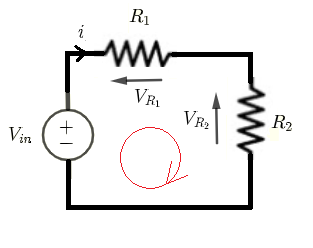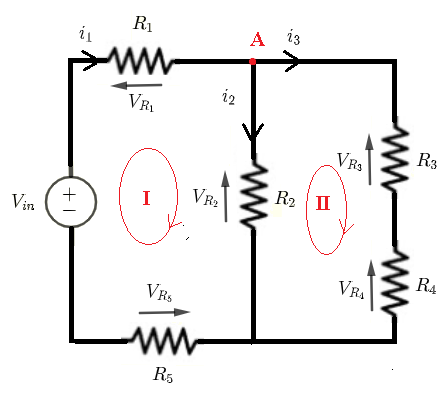# DC Circuits Calculators of Currents and Voltages

  

Three online calculators to calculate all the currents and voltages in three different dc circuit are presented. Practice solving dc circuits manually and check answers using these calculators.

## 1 - Voltages and Currents Formulas in Circuit 1Given $V_{in}$ , $R_1$ and $R_2$, let us find formulas for the currents through and the voltages across all resistors.
Using Kirchhoff's laws of voltages to write the equations:
$V_{in} = R_1 i + R_2 i$    (I)
Solve for $i$
$i = \dfrac{V_{in}}{R_1 + R_2}$
Use Ohm's law to find the voltages
$V_{R_1} = i R_{1} = \dfrac{ R_{1} V_{in}}{R_1 + R_2}$
$V_{R_2} = i R_{2} = \dfrac{ R_{2} V_{in}}{R_1 + R_2}$

### Voltages and Currents Calculator for Circuit 2

Enter the voltage source $V_{in}$ in volts and the resistors $R_1$ and $R_4$ in $\Omega$ and press "Calculate". The calculator uses the above formulas to calculates all currents and voltages whose formulas were obtained above.

 $R_1$ = 10 $\Omega$       $R_2$ = 20 $\Omega$ $V_{in}$ = 12 V Decimal Places = 4

## 2 - Voltages and Currents Formulas in Circuit 2Given $V_{in}$ , $R_1$, $R_2$, $R_3$ and $R_4$, let us find formulas for the currents through and the voltages across all resistors.
Using Kirchhoff's laws of voltages to write the equations:
Loop (I): $V_{in} = R_1 i_1 + R_2 i_2$     (I)
Loop (II): $- R_2 i_2 + R_3 i_3 + R_4 i_3 = 0$     (II)
Using Kirchhoff's laws of currents to write the equation:
Node (A): $i_1 = i_2 + i_3$     (III)
Substitute $i_1$ by $i_2 + i_3$ in equations (I) and rearrange equations (I) and (II) to have a system in two equations and two unknowns $i_2$ and $i_3$
$(R_1 + R_2) i_2 + R_1 i_3 = V_{in}$
$- R_2 i_2 + (R_3 + R_4) i_3 = 0$
Use any method to solve the above system of equations to obtain
$i_2 = \dfrac{R_3 + R_4}{(R_1 + R_2)(R_3+R_4)+R_1R_2} V_{in}$
$i_3 = \dfrac{R_2}{(R_1 + R_2)(R_3+R_4)+R_1R_2} V_{in}$
Use equation (III) to find $i_1$
$i_1 = i_2+i_3 = \dfrac{R_2+R_3 + R_4}{(R_1 + R_2)(R_3+R_4)+R_1R_2} V_{in}$

The voltages across the resistors are given by Ohm's law as follows:
$V_{R_1} = R_1 i_1 = \dfrac{R_1(R_2 + R_3 + R_4)}{(R_1 + R_2)(R_3+R_4)+R_1R_2} V_{in}$

$V_{R_2} = R_2 i_2 = \dfrac{R_2(R_3 + R_4)}{(R_1 + R_2)(R_3+R_4)+R_1R_2} V_{in}$

$V_{R_3} = R_3 i_3 = \dfrac{R_3 R_2}{(R_1 + R_2)(R_3+R_4)+R_1R_2} V_{in}$

$V_{R_4} = R_4 i_3 = \dfrac{R_4 R_2}{(R_1 + R_2)(R_3+R_4)+R_1R_2} V_{in}$

### Voltages and Currents Calculator for Circuit 2

Enter the voltage source $V_{in}$ in volts and the resistors $R_1, R_2, R_3$ and $R_4$ in $\Omega$ and press "Calculate". The calculator uses the above formulas to calculates all currents and voltages whose formulas were obtained above.

 $R_1$ = 10 $\Omega$       $R_2$ = 20 $\Omega$ $R_3$ = 10 $\Omega$       $R_4$ = 20 $\Omega$ $V_{in}$ = 12 V Decimal Places = 4

## 3 - Voltages and Currents Formulas in Circuit 3Given $V_{in}$ , $R_1$, $R_2$, $R_3$ , $R_4$ and $R_5$, let us find formulas for the currents through and the voltages across all resistors.
Using Kirchhoff's laws of voltages to write the equations:
Loop (I): $V_{in} = R_1 i_1 + R_2 i_2 + R_5 i_1$    (I)
Loop (II): $- R_2 i_2 + R_3 i_3 + R_4 i_3 = 0$    (II)
Using Kirchhoff's laws of currents to write the equation:
Node (A): $i_1 = i_2 + i_3$

Equation (I) obtained from Kirchhoff's laws for circuits 2 is given by
$V_{in} = R_1 i_1 + R_2 i_2$
Equation (I) obtained from Kirchhoff's laws for circuits 3 above is given by
$V_{in} = R_1 i_1 + R_2 i_2 + R_5 i_1 = (R_1 + R_5) i_1 + R_2 i_2$
The only difference is that the coefficient of $i_1$ is $R_1 + R_5$ in circuit 3 and $R_1$ in circuit 2. We therefore do need to solve the system of the three equations from start, but we just replace $R_1$ in the solutions for circuit 2 by $R_1 + R_5$ to obtain the solutions for circuit 3 which are given by
$i_1 = \dfrac{R_2+R_3 + R_4}{(R_1 + R_5 + R_2)(R_3+R_4)+(R_1 + R_5) R_2} V_{in}$
$i_2 = \dfrac{R_3 + R_4}{(R_1 + R_5 + R_2)(R_3+R_4)+(R_1 + R_5) R_2} V_{in}$
$i_3 = \dfrac{R_2}{(R_1 + R_5 + R_2)(R_3+R_4)+(R_1 + R_5) R_2} V_{in}$

The voltages across the resistors are given by:
$V_{R_1} = R_1 i_1 = \dfrac{R_1(R_2+R_3 + R_4)}{(R_1 + R_5 + R_2)(R_3+R_4)+(R_1 + R_5) R_2} V_{in}$

$V_{R_2} = R_2 i_2 = \dfrac{R_2(R_3 + R_4)}{(R_1 + R_5 + R_2)(R_3+R_4)+(R_1 + R_5) R_2} V_{in}$

$V_{R_3} = R_3 i_3 = \dfrac{R_3 R_2}{(R_1 + R_5 + R_2)(R_3+R_4)+(R_1 + R_5) R_2} V_{in}$

$V_{R_4} = R_4 i_3 = \dfrac{R_4 R_2}{(R_1 + R_5 + R_2)(R_3+R_4)+(R_1 + R_5) R_2} V_{in}$

$V_{R_4} = R_5 i_1 = \dfrac{R_5(R_2+R_3 + R_4)}{(R_1 + R_5 + R_2)(R_3+R_4)+(R_1 + R_5) R_2} V_{in}$

### Voltages and Currents Calculator for Circuit 3

Enter the voltage source $V_{in}$ in volts and the resistors $R_1, R_2, R_3, R_4$ and $R_5$ in $\Omega$ and press "Calculate". The calculator uses the above formulas to calculates all currents and voltages whose formulas were obtained above.

 $R_1$ = 10 $\Omega$       $R_2$ = 20 $\Omega$ $R_3$ = 30 $\Omega$       $R_4$ = 30 $\Omega$ $R_5$ = 30 $\Omega$ $V_{in}$ = 12 V Decimal Places = 4